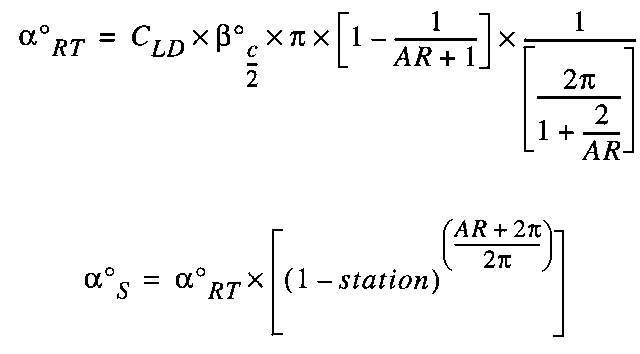# The Culver Twist Formula

Irv Culver was a resident of Playa del Rey, California, and was involved to some extent in tailless aircraft design for six decades. The following information is derived from a paper Irv wrote in 1986 and which was published in the TWITT Newsletter, No. 4, October 1986.

The equations

The Culver equation is formulated to provide a twist distribution which provides a very close approximation to the optimum span loading. In sum, increasing the coefficient of lift near the center line of the wing reduces the necessary down force generated by the wing tips. If you can mold the lift distribution to a more elliptical shape, the induced drag is reduced.

Interestingly, getting the lift distribution close to the ideal elliptical shape requires several control points. Two control points, root and tip, is obviously insufficient for the task, but many control points would make for difficult and time consuming construction. Irv was able to minimize the number of points at three - one at the root, one at the tip, and the third at a point 0.3 of the semi-span out from the center line. Most wings can thus be fashioned with three tamplates when using the foam core method.

There are five variables which influence the amount of required geometric wing twist:
CLD, design coefficient of lift:
The greater the design coefficient of lift, the more wing twist will be required. The design coefficient should be chosen as described above.

AR, aspect ratio:
The aspect ratio of the complete wing.

¦Ī:
The sweep angle of the wing in degrees as measured at the half chord line.

RT:
Total twist angle of the zero lift lines from root to tip.

S:
The angle of the zero lift line at any station relative to the tip (1.0) in degrees.Calculation of required wing twist using the above formulae can be easily accomplished on a hand-held calculator, but we highly recommend Aaron Coffey's Excel/Appleworks spreadsheet. The spreadsheet allows you to see the results of parameter changes nearly instantaneously.

A few caveats and suggestions are in order:
(1) Do not invert airfoils. Use sections with forward camber and low pitching moments.
(2) Twist angles from the formula are always denoted relative to the zero lift angle.
(3) Contrary to the Panknin formula, in which the design coefficient of lift is usually that at cruise, Irv's formula is based on a coefficient of lift between 0.8 and 1.4. Use 0.8 if high speed is the only goal. Coefficients of lift of 1.0 to 1.2 should be used for high performance thermal machines.
(4) The elevons to be used are of tapered planform. At the wing tip, the elevon can be up to 50% of the chord, tapering down to a zero percent chord at around 40% of the semi-span. This allows good thermalling with the elevons in trail, and high speed flight is accomplished using a minimal amount of down trim.

• Aaron Coffey has written an Excel spreadsheet to calculate the required geometric twist when using the Culver twist distribution.
culvercolor.xls.zip; the Culver template, in color,  for Excel.
culvergray.xls.zip; the Culver template, grayscale, for Excel.
culvercolor.cwk.sit; the Culver template, in color,  for Appleworks.
culvergray.cwk.sit; the Culver template, grayscale,  for Appleworks.